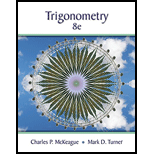Chapter 1.5, Problem 50PSTrigonometry (MindTap Course List)

8th Edition
Charles P. McKeague + 1 other
ISBN: 9781305652224

Solutions

Chapter
SectionTrigonometry (MindTap Course List)

8th Edition
Charles P. McKeague + 1 other
ISBN: 9781305652224
Textbook Problem

Multiply. ( 1 − cos   θ ) ( 1 + cos   θ )

To determine

To multiply the given expression.

Explanation

Given:

The given expression is (1cosθ)(1+cosθ).

Calculation:

(1cosθ)(1+cosθ)=1(1cosθ)+cosθ(1cosθ)                (Distribute the first binomial into the second)=11+1(cosθ)+cosθ1+cosθ(cosθ) <

Still sussing out bartleby?

Check out a sample textbook solution.

See a sample solution

The Solution to Your Study Problems

Bartleby provides explanations to thousands of textbook problems written by our experts, many with advanced degrees!

Get Started

Factor completely: 16y28y+1

Elementary Technical Mathematics

In Problems 11-14, find the particular solution.

Mathematical Applications for the Management, Life, and Social Sciences

[1, 4)

Applied Calculus for the Managerial, Life, and Social Sciences: A Brief Approach

Find dy/dx by implicit differentiation. 8. x3 xy2 + y3 = 1

Single Variable Calculus: Early Transcendentals, Volume I